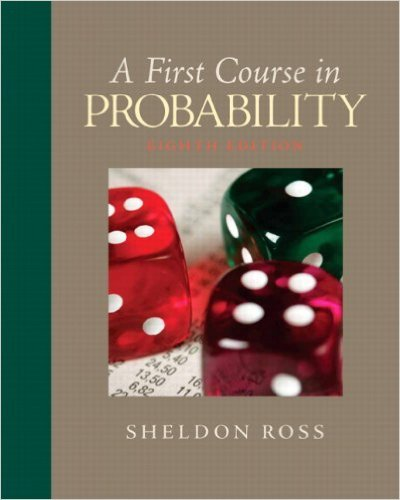×
×

# Solutions for Chapter 3: First Course in Probability 8th Edition## Full solutions for First Course in Probability | 8th Edition

ISBN: 9780136033134Solutions for Chapter 3

Solutions for Chapter 3
4 5 0 339 Reviews
25
5
##### ISBN: 9780136033134

Chapter 3 includes 90 full step-by-step solutions. This textbook survival guide was created for the textbook: First Course in Probability, edition: 8. Since 90 problems in chapter 3 have been answered, more than 6307 students have viewed full step-by-step solutions from this chapter. This expansive textbook survival guide covers the following chapters and their solutions. First Course in Probability was written by and is associated to the ISBN: 9780136033134.

Key Statistics Terms and definitions covered in this textbook
• a-error (or a-risk)

In hypothesis testing, an error incurred by failing to reject a null hypothesis when it is actually false (also called a type II error).

• Asymptotic relative eficiency (ARE)

Used to compare hypothesis tests. The ARE of one test relative to another is the limiting ratio of the sample sizes necessary to obtain identical error probabilities for the two procedures.

• Biased estimator

Unbiased estimator.

• Bivariate normal distribution

The joint distribution of two normal random variables

• Central limit theorem

The simplest form of the central limit theorem states that the sum of n independently distributed random variables will tend to be normally distributed as n becomes large. It is a necessary and suficient condition that none of the variances of the individual random variables are large in comparison to their sum. There are more general forms of the central theorem that allow ininite variances and correlated random variables, and there is a multivariate version of the theorem.

• Conditional probability density function

The probability density function of the conditional probability distribution of a continuous random variable.

• Confounding

When a factorial experiment is run in blocks and the blocks are too small to contain a complete replicate of the experiment, one can run a fraction of the replicate in each block, but this results in losing information on some effects. These effects are linked with or confounded with the blocks. In general, when two factors are varied such that their individual effects cannot be determined separately, their effects are said to be confounded.

• Conidence interval

If it is possible to write a probability statement of the form PL U ( ) ? ? ? ? = ?1 where L and U are functions of only the sample data and ? is a parameter, then the interval between L and U is called a conidence interval (or a 100 1( )% ? ? conidence interval). The interpretation is that a statement that the parameter ? lies in this interval will be true 100 1( )% ? ? of the times that such a statement is made

• Consistent estimator

An estimator that converges in probability to the true value of the estimated parameter as the sample size increases.

• Contour plot

A two-dimensional graphic used for a bivariate probability density function that displays curves for which the probability density function is constant.

• Convolution

A method to derive the probability density function of the sum of two independent random variables from an integral (or sum) of probability density (or mass) functions.

• Correlation coeficient

A dimensionless measure of the linear association between two variables, usually lying in the interval from ?1 to +1, with zero indicating the absence of correlation (but not necessarily the independence of the two variables).

• Counting techniques

Formulas used to determine the number of elements in sample spaces and events.

• Enumerative study

A study in which a sample from a population is used to make inference to the population. See Analytic study

• Error mean square

The error sum of squares divided by its number of degrees of freedom.

• Error sum of squares

In analysis of variance, this is the portion of total variability that is due to the random component in the data. It is usually based on replication of observations at certain treatment combinations in the experiment. It is sometimes called the residual sum of squares, although this is really a better term to use only when the sum of squares is based on the remnants of a model-itting process and not on replication.

• Error variance

The variance of an error term or component in a model.

• Exhaustive

A property of a collection of events that indicates that their union equals the sample space.

• Expected value

The expected value of a random variable X is its long-term average or mean value. In the continuous case, the expected value of X is E X xf x dx ( ) = ?? ( ) ? ? where f ( ) x is the density function of the random variable X.

• Fraction defective control chart

See P chart

×